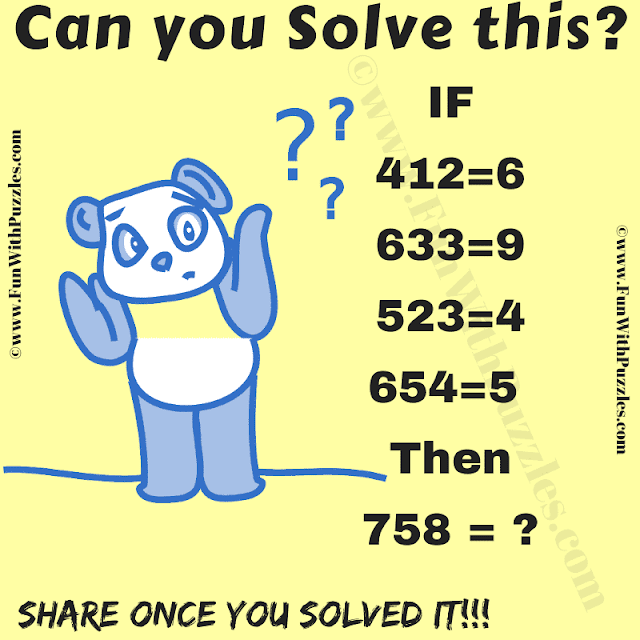This is a hard logic puzzle that you can share with your friends and family members on Whatsapp groups. In this logical reasoning puzzle question, there are some logical number equations. Your challenge in this logic math puzzle is to find the logical reasoning hidden in these number equations. Once you can decipher the hidden logic in this puzzle question, find the value of the missing number.Can you solve this Hard Logic Puzzle?

This is a tough logical reasoning puzzle. If you can solve this puzzle, write down your answer and logical reasoning in the comments box. I will acknowledge the first person to solve this puzzle correctly in this post. Anyway, whether you can solve this puzzle or not, it will be always fun to share this difficult logical puzzle in Whatsapp groups with your friends.

Scorpio said...

5

(a+b+c) - 1, (a+b+c) - 3, (a+b+c) - 6 and so on. The difference number increases in the following pattern 1,3,6,10,15,21 and so on

Rajesh Kumar said...

Thanks for posting your comment and explaining this puzzle logically. However, our logic while creating this puzzle is different from this logic. It will be great if you can think about it again and come up with another answer to this puzzle.

Anonymous said...

Hello,
Could you please confirm if the solution is 5?

Best regards